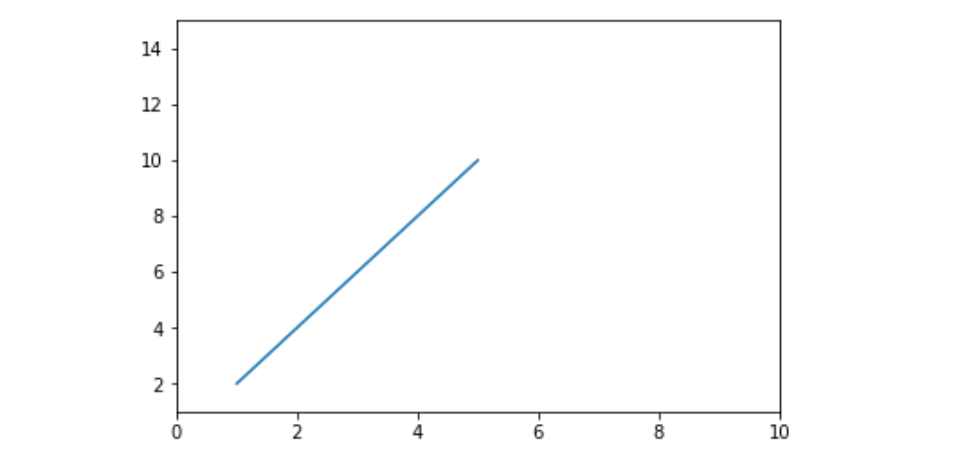Related Articles

# Matplotlib.pyplot.axis() in Python

• Last Updated : 12 Apr, 2020

Matplotlib is a plotting library for creating static, animated, and interactive visualizations in Python. Pyplot is a Matplotlib module which provides a MATLAB-like interface. Matplotlib is designed to be as usable as MATLAB, with the ability to use Python and the advantage of being free and open-source.

## matplotlib.pyplot.axis()

This function is used to set some axis properties to the graph.

Syntax: matplotlib.pyplot.axis(*args, emit=True, **kwargs)

Parameters:
xmin, xmax, ymin, ymax:These parameters can be used to
set the axis limits on the graph
emit:Its a bool value used to notify observers of the axis limit change

Example #1:

 `import` `matplotlib.pyplot as plt`` ` ` ` `x ``=``[``1``, ``2``, ``3``, ``4``, ``5``]``y ``=``[``2``, ``4``, ``6``, ``8``, ``10``]`` ` `# Plotting the graph``plt.plot(x, y)`` ` `# Setting the x-axis to 1-10``# and y-axis to 1-15``plt.axis([``0``, ``10``, ``1``, ``15``])`` ` `# Showing the graph with updated axis``plt.show()`

Output:Example #2:

 `import` `matplotlib.pyplot as plt`` ` `x ``=``[``1``, ``2``, ``3``, ``4``, ``5``]``y ``=``[``2``, ``4``, ``6``, ``8``, ``10``]`` ` `plt.plot(x, y)`` ` `# we can turn off the axis and display``# only the line by passing the ``# optional parameter 'off' to it``plt.axis(``'off'``)`` ` `plt.show()`

Output:Attention geek! Strengthen your foundations with the Python Programming Foundation Course and learn the basics.

To begin with, your interview preparations Enhance your Data Structures concepts with the Python DS Course. And to begin with your Machine Learning Journey, join the Machine Learning – Basic Level Course

My Personal Notes arrow_drop_up